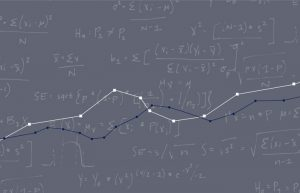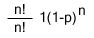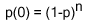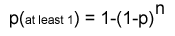# Deriving a Problem Discovery Sample Size

Jeff Sauro, PhDNielsen derives his “five users is enough” formula from a paper he and Tom Landauer published in 1993. Before Nielsen and Landauer James Lewis of IBM proposed a very similar problem detection formula in 1982 based on the binomial probability formula. Lewis stated that:

The binomial probability theorem can be used to determine the probability that a problem of probability p will occur r times during a study with n subjects. For example, if an instruction will be confusing to 50% of the user population, the probability that one subject will be confused is .5.

In 1990 and 1992 Robert Virzi outlined a predicted probability formula 1-(1-p)n where p is the probability of detecting a given problem and n is the sample size. Using the data we have about the Butterfly Ballot example we can derive the sample size using Tog’s value of p (.10) of a user having some confusion about the ballot. If we wanted to have a 90% likelihood of detecting one problem we can solve for the number of users needed with the formula:

.90 (likelihood of detection)= 1-(1-.1) n

Simplifying the equation:

.90 = 1-(.9) n

Then isolating the variable by subtracting 1 from both sides:

.90-1 = -(.9) n

Simplifying again

– .10 = – (.9) n

The negative signs cancel each other out

.10 = .9 n

Solving algebraically for n we multiply both sides of the equation by log.

log(.10) = n(log(.90))

Then divide both sides by log(.90) to isolate n.

n = log(.10) ÷ log(.90)

Finally we arrive at our coveted value of 21.85 or 22 users needed to have a 90% likelihood of detecting this problem once.

Virzi’s formula had a slight derivation when it appeared in the Alertbox column  and with Tom Landauer in the Interchi article which is:

Problems found = N(1-(1-L)n)

Where N is the total number of usability problems in the design, L is the proportion of usability problems discovered while testing a single user and n is the number of users in a test. Nielsen states that the typical value for L is .31. In the Butterfly Ballot example, however, as stated we know the value of L is .10 for this one problem. This lower value of L indicates that this problem is harder to detect than a typical usability problem. Its detection is nonetheless critical in assessing its impact as the subsequent outrage over the election has shown.

Again plugging in the values for the Nielsen and Landauer adjusted formula we get:

90% (Likelihood of Detection) = 1(1-(1-.1) n)

Where N is the 1 problem we’re looking for and L is the .1 likelihood of detection and 90% is the likelihood that at least one user will detect it.

Simplifying the equation again

.90 = 1(1-(.9) n)

We can drop the 1

.90 = 1-.9 n

Subtract 1 from both sides.

.-10 = -.9 n

Again the negatives signs cancel each other out and we take the log of each side.

log(.10) = n(log(.9))

Isolating the n

n= log(.10) ÷ log(.90)

Again we arrive at 21.85. Rounding up to 22 users we would again say that we have a 90% likelihood of detecting the problem once with 22 users.

If we stopped at only the five users as Nielsen recommends, we would only have a 40% probability of seeing that very important problem.

Likelihood of Detection (unknown) = 1(1-(1-.1) 5)

Tog doesn’t tell us where he got the 1 in 10 chance of a user having some trouble with the ballot. We do have a published empirical evaluation showing between .8% and 1.6% of voters failed to cast their correct vote as derived from a statistical analysis of voting in adjacent Florida counties.  You can plug in the approximate value of .01 for L or p and then get the 225 sample size. Lewis published  a quick look-up table for identifying the sample size for identifying a problem once and twice:

Table 1: Sample Size Requirements as a Function of Problem Detection Probability and the Cumulative Likelihood of Detecting the Problem at least Once (Twice) Reprinted with permission from the author.

 Problem Detection Probability Cumulative Likelihood of Detecting the Problem at Least Once (Twice) 0.50 0.75 0.85 0.90 0.95 0.99 0.01 68(166) 136(266) 186(332) 225(382) 289(462) 418(615) 0.05 14(33) 27(53) 37(66) 44(76) 57(91) 82(121) 0.10 7(17) 13(26) 18(33) 22(37) 28(45) 40(60) 0.15 5(11) 9(17) 12(22) 14(25) 18(29) 26(39) 0.25 3(7) 5(10) 7(13) 8(14) 11(17) 15(22) 0.50 1(3) 2(5) 3(6) 4(7) 5(8) 7(10) 0.90 1(2) 1(2) 1(3) 1(3) 2(3) 2(4)

Note: These are the minimum sample sizes that result after rounding cumulative likelihoods to two decimal places. Strictly speaking, therefore, the cumulative probability for the 0.50 column is 0.495, and that for the 0.75 column is 0.745, and so on. If a practitioner requires greater precision, the methods described in the paper will allow the calculation of a revised sample size, which will always be equal to or greater than the sample size in this table. The discrepancy will increase as problem probability decreases, cumulative probability increases, and the number of times a problem must be detected increases.

Using this table
First start with the probability of detecting the usability problem and identify the closest value in the far left column labeled “Problem Detection Probability” (e.g. .01 for a 1% chance, .10 for a 10%). Then identify the percent likelihood of detecting the problem across the top of the columns. You want to have as high a probability as possible as the severity of the problem has nothing to do with the likelihood of occurrence (as the Butterfly Ballot problem clearly shows).

## Deriving Problem Discovery Rates from the Binomial Probability Formula

Although only stated explicitly in Lewis ,,, the binomial probability formula can be used to derive the usability problem discovery formulas also expounded on in Virzi , and Nielsen and Landauer , (Landauer states that they derived their formula from the Poisson Process model, constant probability path independent):When applied to usability problem discovery, n equals the totals number of users, r equals the number of problems and p equals the probability of occurrence. By setting the number of occurrences of a problem r to 0 (zero problems) you get:SimplifiedThe two n! cancel each other out and anything raised to the power of 0 becomes 1. One more level of simplification brings us the probability of detecting zero problems:From this you can derive the probability of detecting at least one problem occurrence by subtracting 1 from the probability of detecting zero problems:If these calculations are too tedious for you there is a calculator that will do it for you.

References

1. Bevan, Nigel; Barnum, Carol; Cockton, Gilbert; Nielsen, Jakob; Spool, Jared; Wixon, Dennis “The “magic number 5″: is it enough for web testing?” in CHI ’03 Extended Abstracts Conference on Human factors in Computing Systems, p.698-699, April 2003
2. Lewis, James “Sample Sizes for Usability Studies: Additional Considerations” in Human Factors 36(2) p. 368-378, 1994
3. Lewis, James “Evaluation of Procedures for Adjusting Problm-Discovery Rates Estimated from Small Samples” in The International Journal of Human-Computer Interaction 13(4) p. 445-479 December 2001
4. Lewis, James “Testing Small System Customer Setup” in Proceedings of the Human Factors Society 26th Annual Meeting p. 718-720 (1982)
5. Molich, R et al. “Comparative Evaluation of Usability Tests.” In CHI 99 Extended Abstracts Conference on Human Factors in Computing Systems, ACM Press, 83-84 1999
6. Nielsen, Jakob and Thomas K. Landauer, “A mathematical model of the finding of usability problems,” Proceedings of the SIGCHI conference on Human factors in computing systems, p.206-213, April 24-29, (1993)
7. Nielsen, Jakob “Why you only need to test with 5 users” Alertbox (2000) http://www.useit.com/alertbox/200319.html
9. Nielsen, Jakob “Probability Theory and Fishing for Significance” Alertbox (2004) Sidebar to Risks of Quantitative Studies http://www.useit.com/alertbox/20040301_probability.html
10. Nielsen, Jakob “Understanding Statistical Significance” Alertbox (2004) Sidebar to Risks of Quantitative Studies http://www.useit.com/alertbox/20040301_significance.html
11. Nielsen, Jakob “Two Sigma: Usability and Six Sigma Quality Assurance” Alertbox (2003) http://www.useit.com/alertbox/20031124.html
12. Nielsen, Jakob and Levy, Jonathan “Measuring Usability: Preference vs. Performance” in Commications of the ACM, Volume 37 p. 66-76 April 1994
13. Spool, J. and Schroeder, W. “Testing Websites: Five Users is Nowhere Near Enough. in CHI 2001 Extended Abstracts, ACM, 285-286 (2001)
14. Tognazzini, Bruce “The Butterfly Ballot: Anatomy of a Disaster” in AskTOG (2001) http://www.asktog.com/columns/042ButterflyBallot.html
15. Virzi, Robert, “Streamlining the design process: Running fewer subjects” in Proceedings of the Human Factors Society 34th Annual Meeting p. 291-294 (1990)
16. Virzi, Robert, “Refining the Test phase of Usability Evaluation: How many subjects is enough?” in Human Factors (34) p 457-468 1992
17. Wand, Jonathan et al “Voting Irregularities in Palm Beach County” November 28, 2001 http://elections.fas.harvard.edu/election2000/palmbeach.pdf,
18. Woolrych, A. and Cockton, G., “Why and When Five Test Users aren’t Enough,” in Proceedings of IHM-HCI 2001 Conference, eds. J. Vanderdonckt, A. Blandford, and A. Derycke, Cépadèus Éditions: Toulouse, Volume 2,105-108, 2001
19. Woolrych, A. and Cockton, G., “Sale must end: should discount methods be cleared off HCI’s shelves?” in Interactions Volume 9, Number 5 (2002)
0
0